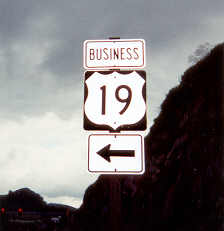# 19

This number is a prime.

Just showing those entries submitted by 'Rivera': (Click here to show all)The smallest sum of three triangular integers equal to a prime: 19 = 3 + 6 + 10. [Rivera]If you plot the seven points (2,3), (3,5), (5,7), (7,11), (11,13), (13,17), (17,19) and calculate the area within this irregular non-convex 7-gon, you will get exactly 19. [Rivera]